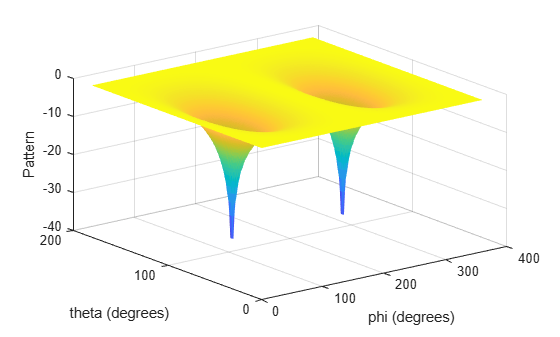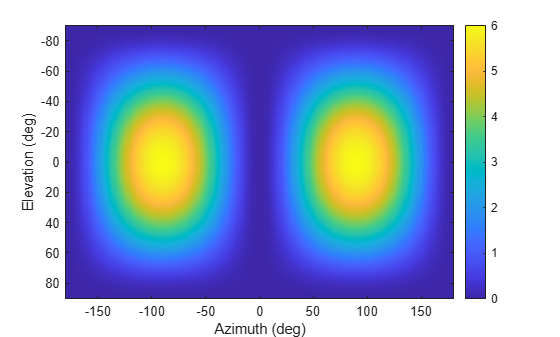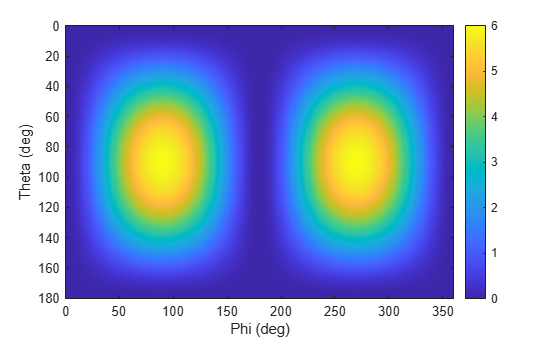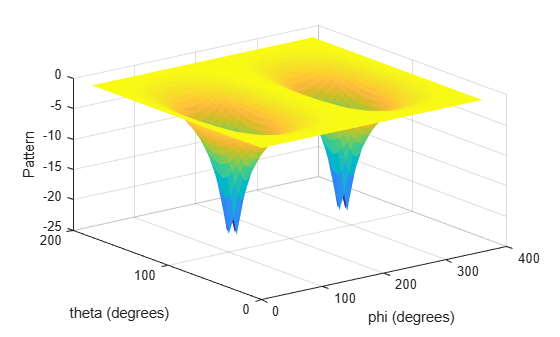# azel2phithetapat

Convert radiation pattern from azimuth-elevation coordinates to phi-theta coordinates

## Syntax

``pat_phitheta = azel2phithetapat(pat_azel,az,el)``
``pat_phitheta = azel2phithetapat(pat_azel,az,el,phi,theta)``
``pat_phitheta = azel2phithetapat(___,'RotateZ2X',rotpatax)``
``[pat_phitheta,phi_pat,theta_pat] = azel2phithetapat(___)``

## Description

example

````pat_phitheta = azel2phithetapat(pat_azel,az,el)` converts the antenna radiation pattern, `pat_azel`, from azimuth and elevation coordinates to the pattern, `pat_phitheta`, in phi and theta coordinates. `az` and `el` are the azimuth and elevation angles at which the `pat_azel` values are defined. The `pat_phitheta` matrix covers theta values from 0 to 180 degrees and phi values from 0 to 360 degrees in one degree increments. The function interpolates the `pat_azel` matrix to estimate the response of the antenna in a given phi-theta direction.```

example

````pat_phitheta = azel2phithetapat(pat_azel,az,el,phi,theta)` also specifies `phi` and `theta` as the grid at which to sample `pat_phitheta`. To avoid interpolation errors, `phi` should cover the range [0,180], and `theta` should cover the range [0,360].```

example

````pat_phitheta = azel2phithetapat(___,'RotateZ2X',rotpatax)` also specifies `rotpatax` to indicate the boresight direction of the pattern along the x-axis or the z-axis.```

example

````[pat_phitheta,phi_pat,theta_pat] = azel2phithetapat(___)` also returns vectors `phi_pat` and `theta_pat` containing the phi and theta angles at which `pat_phitheta` is sampled.```

## Examples

collapse all

Convert a radiation pattern to φ/θ form, with the φ and θ angles spaced 1 degree apart.

Define the pattern in terms of azimuth and elevation.

```az = -180:180; el = -90:90; pat_azel = mag2db(repmat(cosd(el)',1,numel(az)));```

Convert the pattern to φ/θ space.

`pat_phitheta = azel2phithetapat(pat_azel,az,el);`

Plot the result of converting a radiation pattern to $\varphi /\theta$ space with the $\varphi$ and $\theta$ angles spaced 1 degree apart.

The radiation pattern is the cosine of the elevation.

```az = -180:180; el = -90:90; pat_azel = repmat(cosd(el)',1,numel(az));```

Convert the pattern to $\varphi /\theta$ space. Use the returned $\varphi$ and $\theta$ angles for plotting.

`[pat_phitheta,phi,theta] = azel2phithetapat(pat_azel,az,el);`

Plot the result.

```H = surf(phi,theta,mag2db(pat_phitheta)); H.LineStyle = 'none'; xlabel('phi (degrees)'); ylabel('theta (degrees)'); zlabel('Pattern');```Convert a radiation pattern to the alternate phi-theta coordinates, with the phi and theta angles spaced one degree apart.

Create a simple radiation pattern in terms of azimuth and elevation. Add an offset to the pattern to suppress taking the logarithm of zero in `mag2db`.

```az = -180:180; el = -90:90; pat_azel = mag2db(cosd(el).^2'*sind(az).^2 + 1); imagesc(az,el,pat_azel) xlabel('Azimuth (deg)') ylabel('Elevation (deg)') colorbar```Convert the pattern to phi-theta space.

```[pat_phitheta,phi_pat,theta_pat] = azel2phithetapat(pat_azel,az,el,'RotateZ2X',false); imagesc(phi_pat,theta_pat,pat_phitheta) xlabel('Phi (deg)') ylabel('Theta (deg)') colorbar```Convert a radiation pattern to $\varphi /\theta$ space with $\varphi$ and $\theta$ angles spaced 5 degrees apart.

The radiation pattern is the cosine of the elevation.

```az = -180:180; el = -90:90; pat_azel = repmat(cosd(el)',1,numel(az));```

Define the set of $\varphi$ and $\theta$ angles at which to sample the pattern. Then, convert the pattern.

```phi = 0:5:360; theta = 0:5:180; pat_phitheta = azel2phithetapat(pat_azel,az,el,phi,theta);```

Plot the result.

```H = surf(phi,theta,mag2db(pat_phitheta)); H.LineStyle = 'none'; xlabel('phi (degrees)'); ylabel('theta (degrees)'); zlabel('Pattern');```## Input Arguments

collapse all

Antenna radiation pattern as a function of azimuth and elevation, specified as a real-valued Q-by-P matrix. `pat_azel` contains the magnitude pattern. P is the length of the `az` vector, and Q is the length of the `el` vector. Units are in dB.

Data Types: `double`

Azimuth angles at which the `pat_azel` pattern is sampled, specified as a real-valued length-P vector. Azimuth angles lie between –180 and 180, inclusive. Units are in degrees.

Data Types: `double`

Elevation angles at which the `pat_azel` pattern is sampled, specified as a real-valued length-Q vector. Azimuth angles lie between –90 and 90, inclusive. Units are in degrees.

Data Types: `double`

Phi angles at which the `pat_phitheta` pattern is sampled, specified as a real-valued length-L vector. Phi angles lie between 0 and 360, inclusive. Units are in degrees.

Data Types: `double`

Theta angles at which the `pat_phitheta` pattern is sampled, specified as a real-valued length-M vector. Theta angles lie between 0 and 180, inclusive. Units are in degrees.

Data Types: `double`

Pattern boresight direction selector, specified as `true` or `false`.

• If `rotpatax` is `true`, the pattern boresight is along the x-axis. In this case, the z-axis of phi-theta space is aligned with the x-axis of azimuth and elevation space. The phi angle is defined from the y-axis to the z-axis and the theta angle is defined from the x-axis toward the yz-plane. (See Phi and Theta Angles).

• If `rotpatax` is `false`, the phi angle is defined from the x-axis to the y-axis and the theta angle is defined from the z-axis toward the xy-plane. (See Alternative Definition of Phi and Theta).

Data Types: `logical`

## Output Arguments

collapse all

Antenna radiation pattern in phi-theta coordinates, returned as a real-valued M-by-L matrix. `pat_phitheta` represents the magnitude pattern. L is the length of the `phi_pat` vector, and M is the length of the `theta_pat` vector. Units are in dB.

Phi angles at which the `pat_phitheta` pattern is sampled, returned as a real-valued length L vector. Units are in degrees.

Theta angles at which the `pat_phitheta` pattern is sampled, returned as a real-valued length-M vector. Units are in degrees.

collapse all

### Azimuth and Elevation Angles

The azimuth angle of a vector is the angle between the x-axis and the orthogonal projection of the vector onto the xy plane. The angle is positive in going from the x axis toward the y axis. Azimuth angles lie between –180 and 180 degrees. The elevation angle is the angle between the vector and its orthogonal projection onto the xy-plane. The angle is positive when going toward the positive z-axis from the xy plane. By default, the boresight direction of an element or array is aligned with the positive x-axis. The boresight direction is the direction of the main lobe of an element or array.

Note

The elevation angle is sometimes defined in the literature as the angle a vector makes with the positive z-axis. The MATLAB® and Phased Array System Toolbox™ products do not use this definition.

This figure illustrates the azimuth angle and elevation angle for a vector shown as a green solid line.### Phi and Theta Angles

The phi angle (φ) is the angle from the positive y-axis to the vector’s orthogonal projection onto the yz plane. The angle is positive toward the positive z-axis. The phi angle is between 0 and 360 degrees. The theta angle (θ) is the angle from the x-axis to the vector itself. The angle is positive toward the yz plane. The theta angle is between 0 and 180 degrees.

The figure illustrates phi and theta for a vector that appears as a green solid line.The coordinate transformations between φ/θ and az/el are described by the following equations

`$\begin{array}{l}\mathrm{sin}el=\mathrm{sin}\varphi \mathrm{sin}\theta \\ \mathrm{tan}az=\mathrm{cos}\varphi \mathrm{tan}\theta \\ \mathrm{cos}\theta =\mathrm{cos}el\mathrm{cos}az\\ \mathrm{tan}\varphi =\mathrm{tan}el/\mathrm{sin}az\end{array}$`

### Alternative Definition of Phi and Theta

The phi angle (φ) is the angle from the positive x-axis to the vector’s orthogonal projection onto the xy plane. The angle is positive toward the positive y-axis. The phi angle is between 0 and 360 degrees. The theta angle (θ) is the angle from the z-axis to the vector itself. The angle is positive toward the xy plane. The theta angle is between 0 and 180 degrees.

The figure illustrates φ and θ for a vector that appears as a green solid line.`$\begin{array}{l}\varphi =az\\ \theta =90-el\\ az=\varphi \\ el=90-\theta \end{array}$`

## Version History

Introduced in R2012a